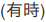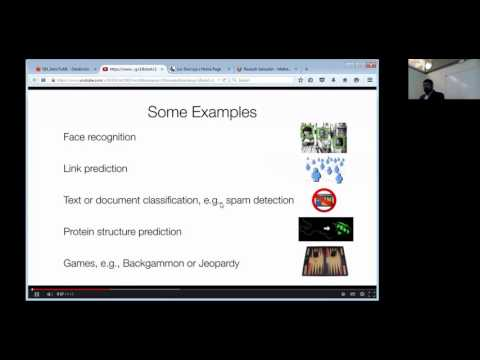// Databricks notebook source exported at Sat, 18 Jun 2016 10:59:31 UTC

# Scalable Data Science

### prepared by Raazesh Sainudiin and Sivanand Sivaram

The html source url of this databricks notebook and its recorded Uji:SOURCE: This is the scala version of the python notebook from the databricks Community Edition that has been added to this databricks shard at Workspace -> scalable-data-science -> xtraResources -> dbCE -> MLlib -> unsupervised -> clustering -> k-means -> 1MSongsPy_ETLExploreModel as extra resources for this project-focussed course Scalable Data Science.

# Stage 3: Modeling Songs via k-meansThis is the third step into our project. In the first step we parsed raw text files and created a table. Then we explored different aspects of data and learned that things have been changing over time. In this step we attempt to gain deeper understanding of our data by categorizing (a.k.a. clustering) our data. For the sake of training we pick a fairly simple model based on only three parameters. We leave more sophisticated modeling as exercies to the reader

We pick the most commonly used and simplest clustering algorithm (KMeans) for our job. The SparkML KMeans implementation expects input in a vector column. Fortunately, there are already utilities in SparkML that can help us convert existing columns in our table to a vector field. It is called `VectorAssembler`. Here we import that functionality and use it to create a new DataFrame

``````
import org.apache.spark.ml.feature.VectorAssembler

val trainingData = new VectorAssembler()
.setInputCols(Array("duration", "tempo", "loudness"))
.setOutputCol("features")
.transform(table("songsTable"))

``````

All we have done above with the `VectorAssembler` method is:

• created a DataFrame called `trainingData`
• that `transform`ed our `table` called `songsTable`
• by adding an output column named `features` using `setOutputCol("features")`
• that was obtained from an `Array` of the `songsTable`’s columns named `duration`, `tempo` and `loudness` using
• `setInputCols(Array("duration", "tempo", "loudness"))`.
``````
trainingData.take(3) // see first 3 rows of trainingData DataFrame, notice the vectors in the last column

``````

### Transformers

A Transformer is an abstraction that includes feature transformers and learned models. Technically, a Transformer implements a method transform(), which converts one DataFrame into another, generally by appending one or more columns. For example:

• A feature transformer might take a DataFrame, read a column (e.g., text), map it into a new column (e.g., feature vectors), and output a new DataFrame with the mapped column appended.
• A learning model might take a DataFrame, read the column containing feature vectors, predict the label for each feature vector, and output a new DataFrame with predicted labels appended as a column.

### Estimators

An Estimator abstracts the concept of a learning algorithm or any algorithm that fits or trains on data.

Technically, an Estimator implements a method `fit()`, which accepts a DataFrame and produces a Model, which is a Transformer.

For example, a learning algorithm such as `LogisticRegression` is an Estimator, and calling `fit()` trains a `LogisticRegressionModel`, which is a Model and hence a Transformer.

``````
display(trainingData.select("duration", "tempo", "loudness", "features").limit(5)) // see features in more detail

``````

#### Demonstration of the standard algorithm

(1)(2)(3)(4)1. k initial “means” (in this case k=3) are randomly generated within the data domain (shown in color).
• k clusters are created by associating every observation with the nearest mean. The partitions here represent the Voronoi diagram generated by the means.
• The centroid of each of the k clusters becomes the new mean.
• Steps 2 and 3 are repeated until local convergence has been reached.

The “assignment” step 2 is also referred to as expectation step, the “update step” 3 as maximization step, making this algorithm a variant of the generalized expectation-maximization algorithm.

Caveats: ** As k-means is a **heuristic algorithm, there is no guarantee that it will converge to the global optimum, and the result may depend on the initial clusters. As the algorithm is usually very fast, it is common to run it multiple times with different starting conditions. However, in the worst case, k-means can be very slow to converge. For more details see https://en.wikipedia.org/wiki/K-means_clustering that is also embedded in-place below.

``````
//This allows easy embedding of publicly available information into any other notebook
//when viewing in git-book just ignore this block - you may have to manually chase the URL in frameIt("URL").
//Example usage:
// displayHTML(frameIt("https://en.wikipedia.org/wiki/Latent_Dirichlet_allocation#Topics_in_LDA",250))
def frameIt( u:String, h:Int ) : String = {
"""<iframe
src=""""+ u+""""
width="95%" height="""" + h + """"
sandbox>
<p>
<a href="http://spark.apache.org/docs/latest/index.html">
Fallback link for browsers that, unlikely, don't support frames
</a>
</p>
</iframe>"""
}
displayHTML(frameIt("https://en.wikipedia.org/wiki/K-means_clustering#Standard_algorithm",500))

``````

### CAUTION!

Iris flower data set, clustered using

• k-means (left) and
• true species in the data set (right).k-means clustering result for the Iris flower data set and actual species visualized using ELKI. Cluster means are marked using larger, semi-transparent symbols.

Note that k-means is non-determinicstic, so results vary. Cluster means are visualized using larger, semi-transparent markers. The visualization was generated using ELKI.

With some cautionary tales we go ahead with applying k-means to our dataset next.

We can now pass this new DataFrame to the `KMeans` model and ask it to categorize different rows in our data to two different classes (`setK(2)`). We place the model in a immutable `val`ue named `model`.

Note: This command performs multiple spark jobs (one job per iteration in the KMeans algorithm). You will see the progress bar starting over and over again.

``````
import org.apache.spark.ml.clustering.KMeans
val model = new KMeans().setK(2).fit(trainingData)

``````
``````
//model. // uncomment and place cursor next to . and hit Tab to see all methods on model

``````
``````
model.clusterCenters // get cluster centres

``````
``````
val modelTransformed = model.transform(trainingData) // to get predictions as last column

``````

Remember that ML Pipelines works with DataFrames. So, our trainingData and modelTransformed are both DataFrames

``````
trainingData.printSchema

``````
``````
modelTransformed.printSchema

``````
• The column `features` that we specified as output column to our `VectorAssembler` contains the features
• The new column `prediction` in modelTransformed contains the predicted output
``````
val transformed = modelTransformed.select("duration", "tempo", "loudness", "prediction")

``````

To comfortably visualize the data we produce a random sample. Remember the `display()` function? We can use it to produce a nicely rendered table of transformed DataFrame.

``````
display(transformed.sample(false, fraction = 0.005))

``````

To generate a scatter plot matrix, click on the plot button bellow the table and select `scatter`. That will transform your table to a scatter plot matrix. It automatically picks all numeric columns as values. To include predicted clusters, click on `Plot Options` and drag `prediction` to the list of Keys. You will get the following plot. On the diagonal panels you see the PDF of marginal distribution of each variable. Non-diagonal panels show a scatter plot between variables of the two variables of the row and column. For example the top right panel shows the scatter plot between duration and loudness. Each point is colored according to the cluster it is assigned to.

``````
display(transformed.sample(false, fraction = 0.1)) // try fraction=1.0 as this dataset is small

``````

Do you see the problem in our clusters based on the plot?

As you can see there is very little correlation between loudness, and tempo and generated clusters. To see that, focus on the panels in the first and second columns of the scatter plot matrix. For varying values of loudness and tempo prediction does not change. Instead, duration of a song alone predicts what cluster it belongs to. Why is that?

To see the reason, let’s take a look at the marginal distribution of duration in the next cell.

To produce this plot, we have picked histogram from the plots menu and in `Plot Options` we chose prediction as key and duration as value. The histogram plot shows significant skew in duration. Basically there are a few very long songs. These data points have large leverage on the mean function (what KMeans uses for clustering).

``````
display(transformed.sample(false, fraction = 1.0).select("duration", "prediction")) // plotting over all results

``````

There are known techniques for dealing with skewed features. A simple technique is applying a power transformation. We are going to use the simplest and most common power transformation: logarithm.

In following cell we repeat the clustering experiment with a transformed DataFrame that includes a new column called `log_duration`.

``````
val df = table("songsTable").selectExpr("tempo", "loudness", "log(duration) as log_duration")
val trainingData2 = new VectorAssembler().
setInputCols(Array("log_duration", "tempo", "loudness")).
setOutputCol("features").
transform(df)
val model2 = new KMeans().setK(2).fit(trainingData2)
val transformed2 = model2.transform(trainingData2).select("log_duration", "tempo", "loudness", "prediction")
display(transformed2.sample(false, fraction = 0.1))

``````

The new clustering model makes much more sense. Songs with high tempo and loudness are put in one cluster and song duration does not affect song categories.

Updated: